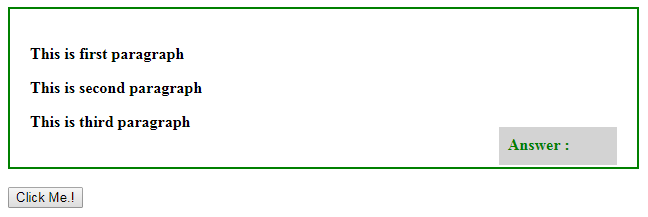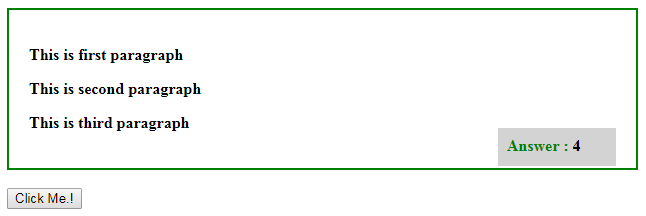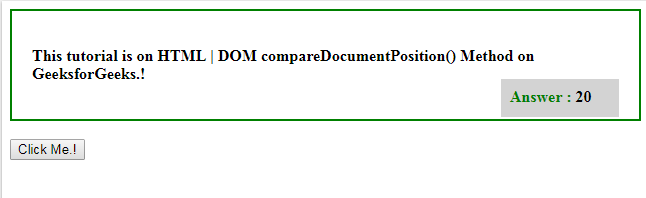Related Articles
HTML | DOM compareDocumentPosition() Method
• Last Updated : 25 Jul, 2019

The DOM compareDocumentPosition() method is used to compare two nodes and it returns an integer describing where they are positioned in the document.

Syntax:

```node1.compareDocumentPosition(node2)
```

Return Value : This return a integer value and their meaning as follows :

• 1: This means that the two nodes do not belong to the same document.
• 2: This means that the two nodes node1 is positioned after node2.
• 4: This means that the two nodes node1 is positioned before node2.
• 8: This means that the two nodes node1 is positioned inside node2.
• 16: This means that the two nodes node2 is positioned inside node1.
• 32: This means that the two nodes have no relationship or they are two attributes on the same element.

Example-1: This will return only single value.

 ```<``html``>``<``style``>``    ``div {``        ``width: 90%;``        ``height: 60%;``        ``padding: 20px;``        ``border: 2px solid green;``        ``font-weight: bold;``    ``}``     ` `    ``#ans {``        ``background-color: lightgrey;``        ``display: block;``        ``width: 100px;``        ``font-weight: bold;``        ``height: 20px;``        ``padding: 9px;``        ``color: green;``        ``float: right;``        ``margin-top: -20px;``    ``}``     ` `    ``#res {``        ``color: black;``    ``}```` ` `<``body``>``    ``<``div``>``        ``<``p` `id``=``"p1"``>``          ``This is first paragraph``        ````       ` `        ``<``p` `id``=``"p2"``>``          ``This is second paragraph``        ````       ` `        ``<``p` `id``=``"p3"``>``          ``This is third paragraph``        ````       ` `        ``<``p` `id``=``"ans"``>Answer : <``span` `id``=``"res"``>``    ````    ``<``br``>``   ` `    ``<``input` `type` `= ``button` `           ``onclick``=``"myFunction()"` `value` `= ``           ``"Click Me.!"` `/>``    ``<``br``>`` ` `    ``<``script``>``        ``function myFunction() {``            ``var x = p1.compareDocumentPosition(p2);``            ``document.getElementById("res").innerHTML = x;``        ``}``    ```` ` ``` ` ``

Output:
Before click on the Button:After click on the button:Example-2: This will return combination of two values.

 ```<``html``>``<``style``>``    ``div {``        ``width: 90%;``        ``height: 60%;``        ``padding: 20px;``        ``border: 2px solid green;``        ``font-weight: bold;``    ``}``     ` `    ``#ans {``        ``background-color: lightgrey;``        ``display: block;``        ``width: 100px;``        ``font-weight: bold;``        ``height: 20px;``        ``padding: 9px;``        ``color: green;``        ``float: right;``        ``margin-top: -20px;``    ``}``     ` `    ``#res {``        ``color: black;``    ``}```` ` `<``body``>``    ``<``div``>``       ` `        ``<``p` `id``=``"p1"``>This tutorial is on ``          ``<``span` `id``=``"p2"``>``             ``HTML | DOM compareDocumentPosition() Method``          `` on GeeksforGeeks.!``        ````       ` `        ``<``p` `id``=``"ans"``>``          ``Answer : ``          ``<``span` `id``=``"res"``>``        ````       ` `    ````    ``<``br``>``   ` `    ``<``input` `type``=``button` `onclick``=``"myFunction()"` `           ``value``=``"Click Me.!"` `/>``    ``<``br``>`` ` `    ``<``script``>``        ``function myFunction() {``            ``var x = p1.compareDocumentPosition(p2);``            ``document.getElementById("res").innerHTML = x;``        ``}``    ```` ` ``` ` ``

Output:
Before Click on the Button:After Click on the Button: Answer will be 20. ‘4’ means that first node is positioned before the second node and ’16’ second node is positioned inside the first node.Note: The return value can be a combination of values. i.e. if the return value is 20 that means p2 is inside p1, ’16’ and p1 is positioned before p2 ‘4’.

Supported Browsers:

• Google Chorme
• Internet Explorer
• Firefox
• Opera
• SafariMy Personal Notes arrow_drop_up